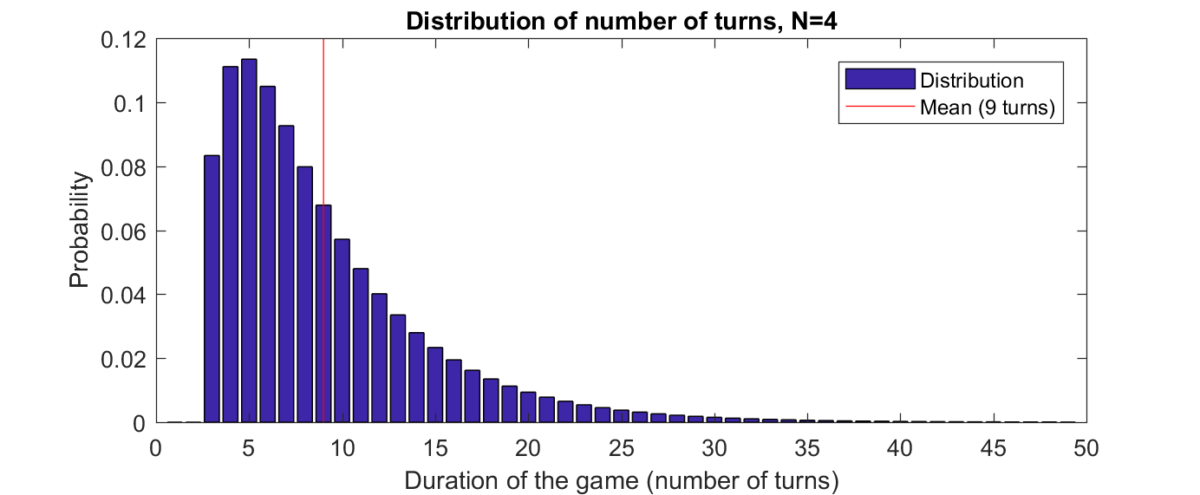# Colorful balls puzzleThis Riddler puzzle about an interesting game involving picking colored balls out of a box. How long will the game last?

You play a game with four balls: One ball is red, one is blue, one is green and one is yellow. They are placed in a box. You draw a ball out of the box at random and note its color. Without replacing the first ball, you draw a second ball and then paint it to match the color of the first. Replace both balls, and repeat the process. The game ends when all four balls have become the same color. What is the expected number of turns to finish the game?

Extra credit: What if there are more balls and more colors?

Here is my solution to the first part (four balls):
[Show Solution]

Here is my solution to the general case with $N$ balls:
[Show Solution]

## 3 thoughts on “Colorful balls puzzle”

1.Stephen Meskin says:

I’m relieved to see 9 which I got doing essentially the same thing by the more primitive method of directly calculating the powers of A and summing multiples of the (5,1) entries, only for me they were the (1,4) entries since I used the transpose and I eliminated state (1,1,1,1). I like your trick of setting the (5,5) entry of A to zero. I set the corresponding entry to 1 and had to subtract before summing.

2.NM Solver says:

Since we know mean of E1 dstribution if we can calc mode (max prob) we can fit the lognormal. How to do that?

3.Peter Calhoun says:

Great post! I also used Markov chains to solve the first part, but never knew about the trick where you set all the current absorbing state probabilities to 0.

One minor suggestion is to fully explain the $A_{k+1,k}$ equation:
\begin{align}
\mathbb{P}(k \to k+1, \textrm{Blue Wins}) &= \mathbb{P}(k \to k+1) \cdot \mathbb{P}(\textrm{Blue Wins} \mid k \to k+1) \\
&= \frac{N-k}{N} \frac{k}{N-1} \cdot \frac{k+1}{N}
\end{align}

Perhaps others saw this easily, but it took me a bit of time to see this result. When I increased N, I also found the distribution tends to the log-normal. I agree with your conjecture